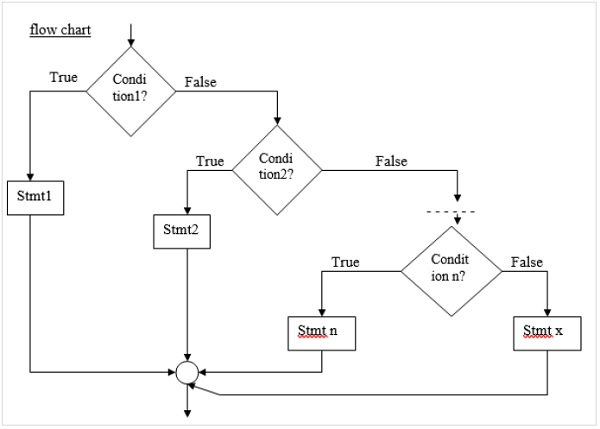# How to use ‘else if ladder’ conditional statement is C language?

Else − if the ladder is the most general way of writing a multi-way decision.

The syntax for else if the ladder is as follows −

if (condition1)
stmt1;
else if (condition2)
stmt2;
- - - - -
- - - - -
else if (condition n)
stmtn;
else
stmt x;

## Flowchart

Refer the flowchart given below −## Example

Following is the C program to execute Else If Ladder conditional statement −

Live Demo

#include<stdio.h>
void main (){
int a,b,c,d;
printf("Enter the values of a,b,c,d: ");
scanf("%d%d%d%d",&a,&b,&c,&d);
if(a>b){
printf("%d is the largest",a);
}
else if(b>c){
printf("%d is the largest",b);
}
else if(c>d){
printf("%d is the largest",c);
}
else{
printf("%d is the largest",d);
}
}

## Output

When the above program is executed, it produces the following result −

Enter the values of a,b,c,d: 2 3 4 5
5 is the largest